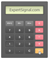Standard Deviation

(SD or 'σ')

Usage: Measuring Volatility

Standard Settings: 20/21 PeriodsIntroduction To Standard Deviation

Standard deviation is a very popular statistical tool that can be used for measuring market volatility.

-The Standard Deviation's symbol is the Greek letter sigma (σ)

-Standard distribution provides the basis that every pattern gravitates to over time (or else the shrink of values around an average)

Meaning of Standard Deviation:

□ Larger the standard deviation larger the variability of values

□ Smaller the standard deviation smaller the variability of valuesCalculating the Standard Deviation

Standard Deviation can be calculated as follows:

(1) Calculate the average (mean) value of the number of periods

(2) The mean is subtracted from all numbers, and the result is squared

(3) The squared deviations are summed

(4) The total sum is divided by the number of periods

(5) The standard deviation (σ) equals to the square root of the total sumTrading with Standard Deviation

The standard deviation should not be used as an independent system of generating trading signals, instead, it should be used for:

(i) forecasting potential price reversals (based on the principle of reversion to the mean over time)

(ii) confirming the indications of other technical analysis tools, and especially as concerns indications for price reversals

(iii) calculating market risk and adjusting money management accordinglyPlatform Setup

□ GO TO → INDICATORS → TREND → STANDARD DEVIATION

□ SETTINGS → 20/21 PERIODS

■ What is Standard Deviation?

ExpertSignal.com